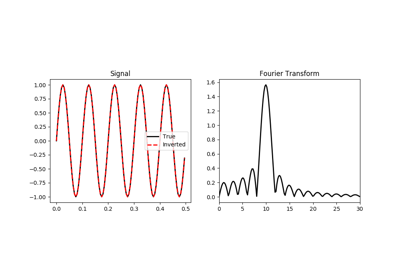# pylops.signalprocessing.FFTND¶

class pylops.signalprocessing.FFTND(dims, dirs=(0, 1, 2), nffts=(None, None, None), sampling=(1.0, 1.0, 1.0), dtype='complex128')[source]

N-dimensional Fast-Fourier Transform.

Apply n-dimensional Fast-Fourier Transform (FFT) to any n axes of a multi-dimensional array depending on the choice of dirs. Note that the FFTND operator is a simple overload to the numpy numpy.fft.fftn in forward mode and to the numpy numpy.fft.ifftn in adjoint mode, however scaling is taken into account differently to guarantee that the operator is passing the dot-test.

Parameters: dims : tuple Number of samples for each dimension dirs : tuple, optional Directions along which FFTND is applied nffts : tuple, optional Number of samples in Fourier Transform for each direction (same as input if nffts=(None, None, None, ..., None)) sampling : tuple, optional Sampling steps in each direction dtype : str, optional Type of elements in input array ValueError If dims, dirs, nffts, or sampling have less than three elements and if the dimension of dirs, nffts, and sampling is not the same

Notes

The FFTND operator applies the n-dimensional forward Fourier transform to a multi-dimensional array. Without loss of generality we consider here a three-dimensional signal $$d(z, y, x)$$. The FFTND in forward mode is:

$D(k_z, k_y, k_x) = \mathscr{F} (d) = \int \int d(z,y,x) e^{-j2\pi k_zz} e^{-j2\pi k_yy} e^{-j2\pi k_xx} dz dy dx$

Similarly, the three-dimensional inverse Fourier transform is applied to the Fourier spectrum $$D(k_z, k_y, k_x)$$ in adjoint mode:

$d(z, y, x) = \mathscr{F}^{-1} (D) = \int \int D(k_z, k_y, k_x) e^{j2\pi k_zz} e^{j2\pi k_yy} e^{j2\pi k_xx} dk_z dk_y dk_x$

Both operators are effectively discretized and solved by a fast iterative algorithm known as Fast Fourier Transform.

Attributes: shape : tuple Operator shape explicit : bool Operator contains a matrix that can be solved explicitly (True) or not (False)

Methods

 __init__(self, dims[, dirs, nffts, …]) Initialize this LinearOperator. adjoint(self) Hermitian adjoint. cond(self, \*\*kwargs_eig) Condition number of linear operator. conj(self) Complex conjugate operator div(self, y[, niter]) Solve the linear problem $$\mathbf{y}=\mathbf{A}\mathbf{x}$$. dot(self, x) Matrix-matrix or matrix-vector multiplication. eigs(self[, neigs, symmetric, niter]) Most significant eigenvalues of linear operator. matmat(self, X) Matrix-matrix multiplication. matvec(self, x) Matrix-vector multiplication. rmatvec(self, x) Adjoint matrix-vector multiplication. transpose(self) Transpose this linear operator.

## Examples using pylops.signalprocessing.FFTND¶Fourier Transform